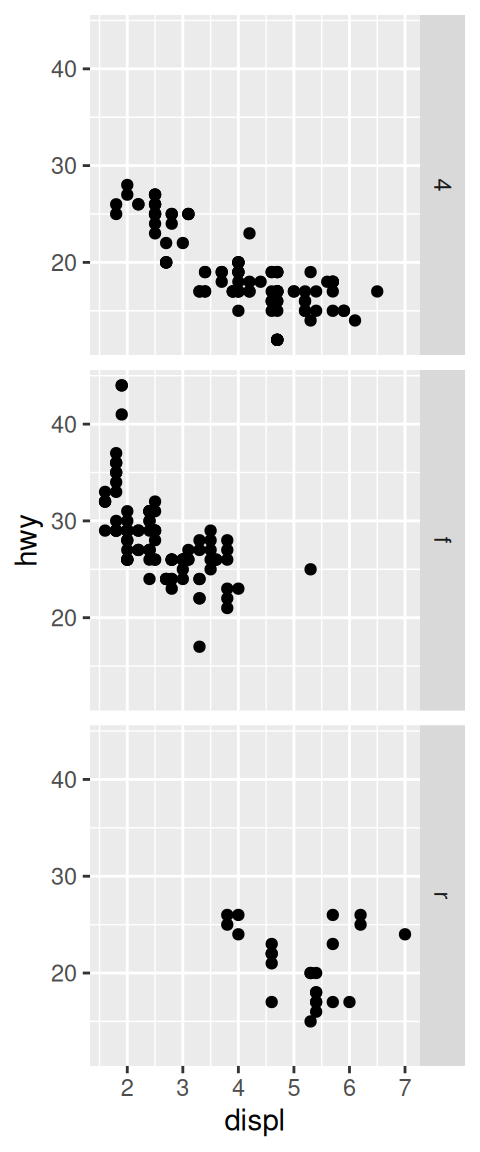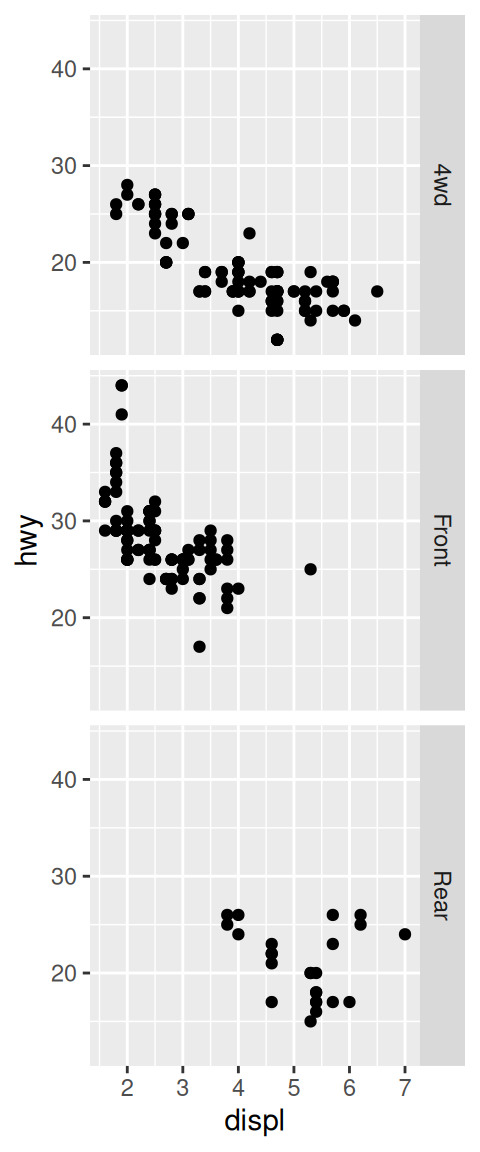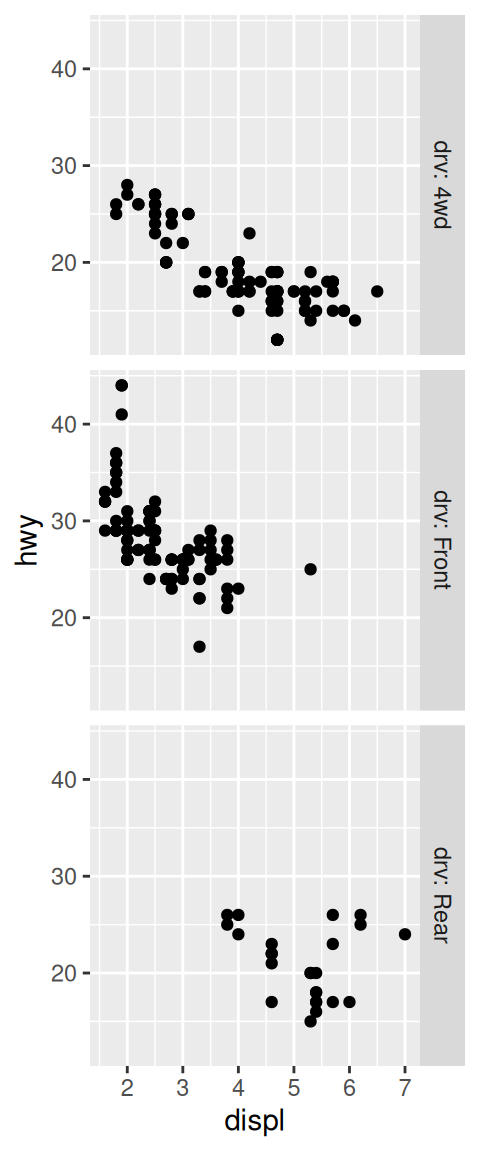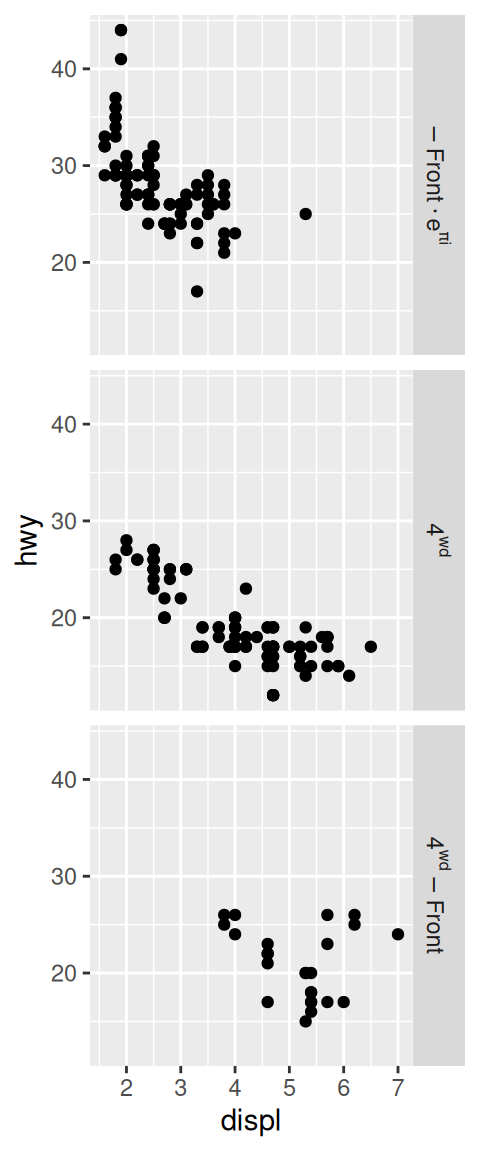## 11.3 Changing the Text of Facet Labels

### 11.3.1 Problem

You want to change the text of facet labels.

### 11.3.2 Solution

Change the names of the factor levels (Figure 11.4):

``````library(dplyr)

# Make a modified copy of the original data
mpg_mod <- mpg %>%
# Rename 4 to 4wd, f to Front, r to Rear
mutate(drv = recode(drv, "4" = "4wd", "f" = "Front", "r" = "Rear"))

# Plot the new data
ggplot(mpg_mod, aes(x = displ, y = hwy)) +
geom_point() +
facet_grid(drv ~ .)``````Figure 11.4: Default facet labels (left); Modified facet labels (right)

### 11.3.3 Discussion

Unlike with scales where you can set the labels, to set facet labels you must change the data values. Also, at the time of this writing, there is no way to show the name of the faceting variable as a header for the facets, so it can be useful to use descriptive facet labels.

With `facet_grid()` but not `facet_wrap()`, at this time), it’s possible to use a labeller function to set the labels. The labeller function `label_both()` will print out both the name of the variable and the value of the variable in each facet (Figure 11.5, left):

``````ggplot(mpg_mod, aes(x = displ, y = hwy)) +
geom_point() +
facet_grid(drv ~ ., labeller = label_both)``````

Another useful labeller is label_parsed(), which takes strings and treats them as R math expressions (Figure 11.5, right):

``````# Make a modified copy of the original data
mpg_mod <- mpg %>%
mutate(drv = recode(drv,
"4" = "4^{wd}",
"f" = "- Front %.% e^{pi * i}",
"r" = "4^{wd} - Front"
))

ggplot(mpg_mod, aes(x = displ, y = hwy)) +
geom_point() +
facet_grid(drv ~ ., labeller = label_parsed)``````Figure 11.5: With `label_both()` (left); With `label_parsed()` for mathematical expressions (right)